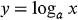1
78
views
33b
Problem

Problem 33b

Chapter 1: Functions and ModelsTextbook ExpertVerified Tutor
18 Oct 2021

Given information

The logarithmic function is provided below.Step-by-step explanation

Step 1.

Concept of logarithmic function

The logarithmicis the inverse function of exponential function in mathematics.

That is, the logarithm of a given number x is the exponent to which another fixed number, the base b, must be raised in order to obtain that number x

The logarithm counts the number of times the same factor appears in repeated multiplication in the simplest instance.